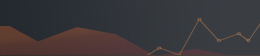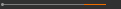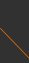﻿ NCTSSponsored by[ Events ]

﻿
Activity Search
Sort out
 select all Seminars and Talks Conference and Workshop Lectures and Courses ElseField
 select all Number Theory and Representation Theory Algebraic Geometry Differential Geometry and Geometrical Analysis Differential Equations and Stochastic Analysis Scientific Computing Interdisciplinary Studies Laboratory of Data Science Harmonic Analysis OthersYear
 select 2015 2016 2017 2018 2019 2020Kinetic Equations and Related Topics week 1-11 (NCKU)Tue. 15:10~17:00 and Thu. 11:10~12:00, September 10 - November 23, 2018 , TBA Speaker: Kung-Chien Wu (National Cheng Kung University & NCTS) Kazuo Aoki (NCTS & National Cheng Kung University) Organizers: Chun-Hsiung Hsia (National Taiwan University) 1.Background and purpose： The main purposes of this course are (1) to construct the quantitative pointwise estimate of the linearized Boltzmann equation with hard sphere case and (2) to give an understanding of the relation between the Boltzmann equation and the fluid-dynamic equations including their boundary conditions.   2.Outline and Speakers： Instructor: Kung-Chien Wu (a) Course Outline: brief introduction to kinetic theory, linearized collision operator, quantitative pointwise estimate of the linearized Boltzmann equation in hard sphere, regularization estimate. (b) Prerequisite Course(s): Real analysis and PDE (c) Grading: Student presentation (d) Textbook: T.P. Liu and S.H. Yu, Solving Boltzmann equation, Part I : Green's function, Bull. Inst. Math. Acad. Sin. (N.S.), 6(2011), 151-243. Instructor: Kazuo Aoki (a) Course Outline: boundary conditions for the Boltzmann equation, non-dimensionalization and similarity laws, Chapman-Enskog and Hilbert expansions and fluid-dynamic equations, slip boundary conditions for the compressible Navier-Stokes equations. (b) Prerequisite Course(s): Linear algebra and multi-variable calculus. (c) Grading: Student presentation (c) Textbook: None. [Reference: Y. Sone, Kinetic Theory and Fluid Dynamics (Birkhauser, 2002); Molecular Gas Dynamics: Theory, Techniques, and Applications (Birkhauser, 2007)]   3.Time and place： Venue: week 1 to week 11 live streaming at NCKU  (Broadcasting at R. 519, Astro-Math. Bldg., NTU); week 12 to week 18 live streaming at R. 440, Astro-Math. Bldg., NTU (Broadcasting in NCKU) Contact: murphyyu＠ncts.ntu.edu.tw(C) 2019 National Center for Theoretical Sciences